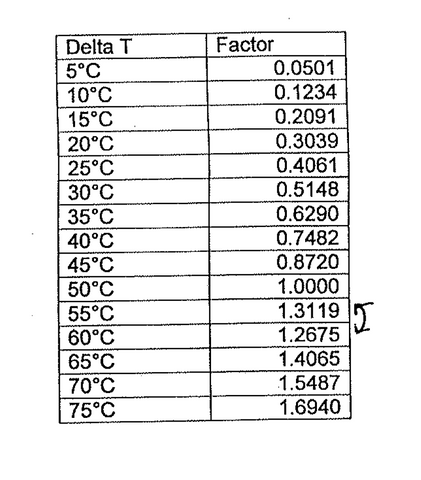# Meaning of Delta 50 (75/65/20)

Meaning of Delta 50 (75/65/20)

Delta 50° (75/65/20) - with Delta T of 50°C, means 75°C inlet water temperature, 65°C outlet water temperature and 20°C of room temperature. The average water temperature in this example is 70°C (i.e. 75° plus 65°, divided by 2).  If we then take the 20°C room temperature we are left with a temperature differential of 50°C or Δt 50°.

Method

Add the flow water temperature and the return temperature together

Subtract the room temperature from the answer this gives you the Delta T temperature

Select the closest Delta T valve from the table and multiply the corresponding factor by the output provided for the particular radiator to obtain the output for your calculated Delta T valve.

If we then take into account the reduced inlet temperature eg you will be getting a heat source pump.
If we replace this with inlet water at 45°C inlet water temperature, 35°C outlet water temperature and 20°C of room temperature. The average water temperature in this example is 40°C (i.e. 45° plus 35°, divided by 2).  If we then take the 20°C room temperature we are left with a temperature differential of 20°C or Δt 20°.

The conversion rate from T50 to T20 is 0.3039.

Eg a radiator 1800 x 500 has 795 Watts at Delta T50 x 0.3039 is 241 Watts (this is the T20 figure).
If we use another Heat Source Pump,  water temperature leaving our system will be 60 degrees returning at 50 and the Delta figure will be 35 degrees.
Eg at Delta 50 say the radiator has a BTU of 3,500.
To convert this to Delta 35 - 3,500 x 0.6290 is 2,202
If we have the figure of at Delta 35 and we want to convert to Delta 50
2,202 / 0.6290 is 3,500 BTU
 Btu Value T50 T50 to T35 (T50 x 0.6290) Equivalent T50 figure to give the required T35 is T50 / 0.6290 3776 2375 6003 3349 2106 5324 3780 2377 6009 5405 3399 8593 2368 1489 3764 4513 2838 7174 4009 2521 6373 5125 3223 8147 2755 1732 4379